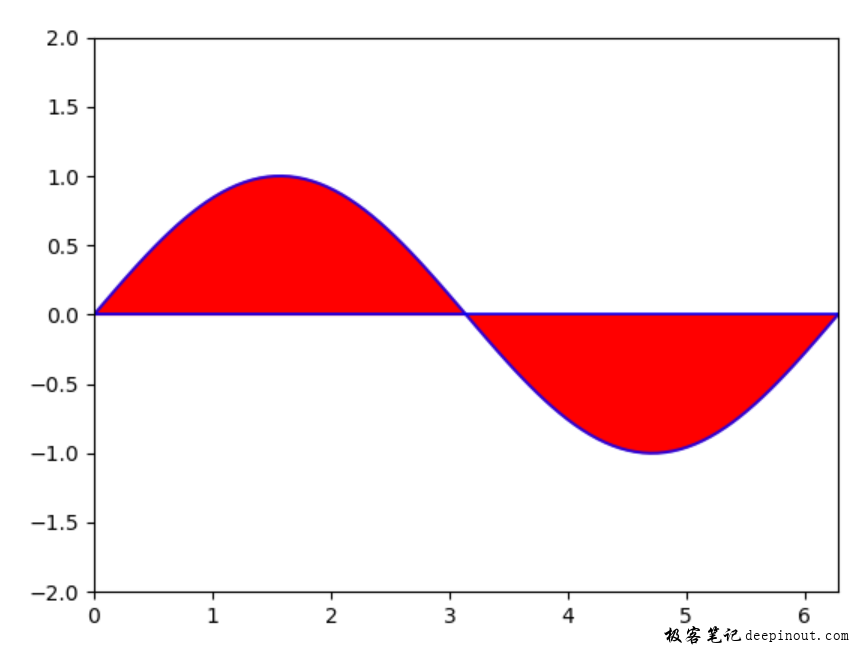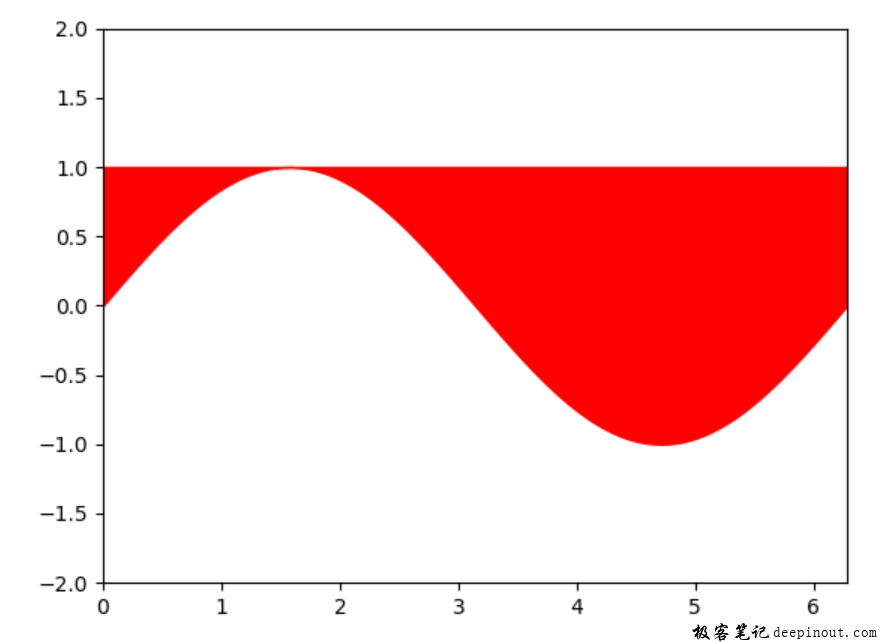# Matplotlib 不规则颜色填充x = np.linspace(0, 2*np.pi, 100)

host.fill(x, y, color = 'r')

host.plot(x, y, color = 'b', alpha = 0.8)

host.plot([x, x[-1]], [y, y[-1]], color = 'b', alpha = 0.8)


import numpy as np
import matplotlib.pyplot as plt

#
fig, host = plt.subplots()  #创建子图
host.grid(False)

host.set_xlim(0, 2*np.pi)
host.set_ylim(-2, 2)

#填充正弦曲线
x = np.linspace(0, 2*np.pi, 100)
y = np.sin(x)

host.fill(x, y, color = 'r')
host.plot(x, y, color = 'b', alpha = 0.8)
host.plot([x, x[-1]], [y, y[-1]], color = 'b', alpha = 0.8)

plt.show()matplotlib.pyplot.fill_between(x, y1, y2=0, where=None, interpolate=False, step=None, *, data=None, **kwargs)

import numpy as np
import matplotlib.pyplot as plt

#
fig, host = plt.subplots()  #创建子图
host.grid(False)

host.set_xlim(0, 2*np.pi)
host.set_ylim(-2, 2)

#填充正弦曲线
x = np.linspace(0, 2*np.pi, 100)
y = np.sin(x)

host.fill_between(x,y, 1, color = 'r')

plt.show()


• 回顶